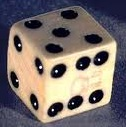# Probability Space: Simple Definition, Examples

Introduction to Probability > Probability Space

## What is a Probability Space?In order to comprehend a statement like “the probability of rolling a die twice and getting two sixes is about 3%”, you need to specify a probability space.
A probability space models random events and is made up of three parts:

1. Sample space: the set of all possible outcomes. For example, if you toss a coin twice, the sample space is {HH,HT,TH,TT}. The sample space is sometimes denoted by the Greek letter omega (Ω).
2. Event space: the set of all events (can be zero to any number). See: What is an event space?
3. Probability function, or the assignment of probability to the event. For example, the odds of tossing a coin and getting a heads is 50%. Probabilities are nonzero and add up to 1.*

Sometimes a definition of a probability space leaves out the event space, leaving just the sample space and the probability. Which of the two definitions is “correct” is open to debate, although the two-item definition is probably easier to work with.

*There are exceptions. For example, if you have items that are not mutually exclusive (i.e. they could both happen at the same time), the probabilities can add up to more than 1. Negative probability only happens in quasiprobability distributions (used in quantum mechanics), which you very likely won’t come across in a basic probability and statistics class.

## Example

Question: You choose a card from a standard deck. What is the probability space for choosing a two?

Solution:
Step 1: Create the sample space. The sample space for this question is a list of all possible cards you could choose (H=hearts, D=diamonds, C=clubs, S=spades):
Ω = {AH,2H,3H,4H,5H,6H,7H,8H,9H,10H,JH,QH,KH, AD,2D,3D,4D,5D,6D,7D,8D,9D,10D,JD,QD,KD, AC,2C,3C,4C,5C,6C,7C,8C,9C,10C,JC,QC,KC, AS,2S,3S,4S,5S,6S,7S,8S,9S,10S,JS,QS,KS}.

Step 2: Assign probabilities. There are 52 choices in the sample space in Step 1. Each card has a 1/52 chance of being chosen.

Note that the question asked for the probability space and not the solution (i.e. it didn’t ask for the “probability of choosing a two.”) You could use the probability space for answering any question though. Just add up the probabilities. For example, the probability of choosing a two would be 1/52 + 1/52 + 1/52 + 1/52 = 4/52 = 1/13.

More examples:

CITE THIS AS:
Stephanie Glen. "Probability Space: Simple Definition, Examples" From StatisticsHowTo.com: Elementary Statistics for the rest of us! https://www.statisticshowto.com/probability-space/
---------------------------------------------------------------------------Need help with a homework or test question? With Chegg Study, you can get step-by-step solutions to your questions from an expert in the field. Your first 30 minutes with a Chegg tutor is free!